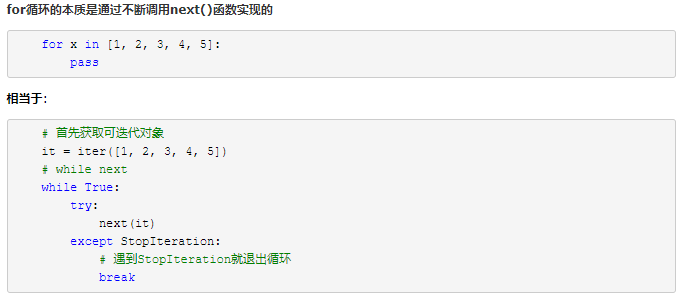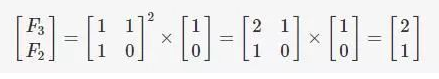### 斐波那契数列之python（5种方法）1、递归方法

``def fib_recur(n):    if n<=1:        return n    else:        return fib_recur(n-1)+fib_recur(n-2) def ex(c):    return int(c) X=input().strip().split()N=list(map(lambda a:int(a), X)) for i in range(1, N):    print(fib_recur(i),end=' ')``

2、递推方法

``def fib_loop(n):    a,b=0,1    for i in range(n+1):        a,b=b,a+b    return a     for i in range(20):    print(fib_loop(i),end=' ')``

3、生成器

``def fib_loop_while(n):    a,b=0,1    for i in range(0,n):        a,b=b,a+b        yield a     for i in fib_loop_while(20):    print(i,end=' ')``

1、带有yield关键字的函数对象 他是返回一个列表list，list中的每个元素 都是 yield返回的每一个值 （每次执行yield输出值构成的列表）

2、带有yield的函数都被看成生成器，生成器是可迭代对象，且具备__iter__ 和 __next__方法， 可以遍历获取元素
python要求迭代器本身也是可迭代的，所以我们还要为迭代器实现__iter__方法，而__iter__方法要返回一个迭代器，迭代器自身正是一个迭代器，所以迭代器的__iter__方法返回自身即可

4、类实现内部魔法方法

``class Fibonacci(object):    """斐波那契数列迭代器"""     def __init__(self,n):        self.n=n        self.a=0        self.b=1        self.cur=0     def __next__(self):        """当使用next()函数调用时，就会获取下一个数"""        if self.cur<self.n:            self.a,self.b=self.b,self.a+self.b            self.cur=self.cur+1            return self.a         else:            raise StopIteration #让他异常停止     def __iter__(self):        """迭代器的__iter__ 返回自身即可"""        return self   if __name__=="__main__":    lst=list(map(int,input().strip().split()))    M=lst        fib=Fibonacci(M)    for n in fib:        print(n,end=' ')    ``5、 矩阵``### 1import numpy as np def fib_matrix(n):    res = pow((np.mat([[1, 1], [1, 0]])), n) * np.mat([, ]) #使用mat方法直接定义矩阵    return res for i in range(10):    print(int(fib_matrix(i)), end=' ')print('\n')  ### 2# 使用矩阵计算斐波那契数列def Fibonacci_Matrix_tool(n):    Matrix = np.matrix([[1, 1], [1, 0]]) #matrix和mat函数等效    # 返回是matrix类型    return pow(Matrix, n)  # pow函数速度快于 使用双星好 ** def Fibonacci_Matrix(n):    result_list = []    for i in range(0, n):        result_list.append(np.array(Fibonacci_Matrix_tool(i)))    return result_list # 调用result=Fibonacci_Matrix(10)for r in result:    print(r,end=' ')``